#### Two-to-one problem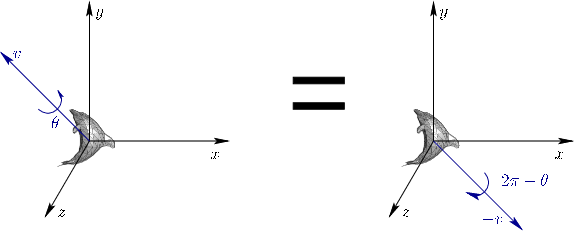Before getting to quaternions, it is important point out one annoying problem with Euler's rotation theorem. As shown in Figure 3.10, it does not claim that the axis-angle representation is unique. In fact, for every 3D rotation other than the identity, there are exactly two representations. This is due to the fact that the axis could point'' in either direction. We could insist that the axis always point in one direction, such as positive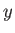, but this does not fully solve the problem because of the boundary cases (horizontal axes). Quaternions, which are coming next, nicely handle all problems with 3D rotations except this one, which is unavoidable.

Quaternions were introduced in 1843 by William Rowan Hamilton. When seeing them the first time, most people have difficulty understanding their peculiar algebra. Therefore, we will instead focus on precisely which quaternions correspond to which rotations. After that, we will introduce some limited quaternion algebra. The algebra is much less important for developing VR systems, unless you want to implement your own 3D rotation library. The correspondence between quaternions and 3D rotations, however, is crucial.

A quaternion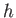is a 4D vector: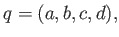(3.28)

in which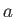,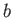,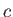, and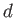can take on real values. Thus,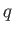can be considered as a point in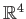. It turns out that we will only use unit quaternions, which means that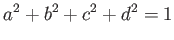(3.29)

must always hold. This should remind you of the equation of a unit sphere (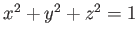), but it is one dimension higher. A sphere is a 2D surface, whereas the set of all unit quaternions is a 3D hypersurface'', more formally known as a manifold [27,153]. We will use the space of unit quaternions to represent the space of all 3D rotations. Both have 3 DOFs, which seems reasonable.

Let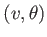be an axis-angle representation of a 3D rotation, as depicted in Figure 3.9. Let this be represented by the following quaternion: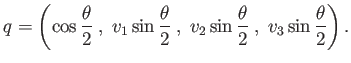(3.30)

Think ofas a data structure that encodes the 3D rotation. It is easy to recoverfrom: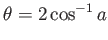and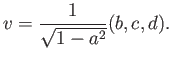(3.31)

If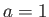, then (3.31) breaks; however, this corresponds to the case of the identity rotation.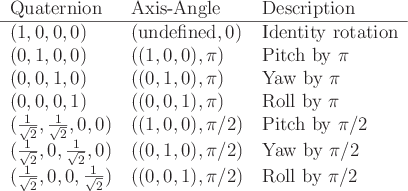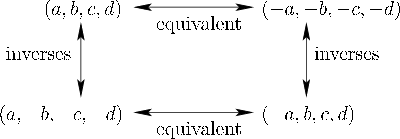You now have the mappings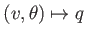and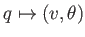. To test your understanding, Figure 3.11 shows some simple examples, which commonly occur in practice. Furthermore, Figure 3.12 shows some simple relationships between quaternions and their corresponding rotations. The horizontal arrows indicate thatand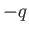represent the same rotation. This is true because of the double representation issue shown in Figure 3.10. Applying (3.30) to both cases establishes their equivalence. The vertical arrows correspond to inverse rotations. These hold because reversing the direction of the axis causes the rotation to be reversed (rotation by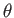becomes rotation by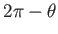).

How do we apply the quaternion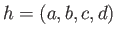to rotate the model? One way is to use the following conversion into a 3D rotation matrix: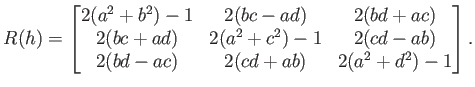(3.32)

A more efficient way exists which avoids converting into a rotation matrix. To accomplish this, we need to define quaternion multiplication. For any two quaternions,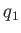and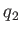, let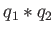denote the product, which is defined as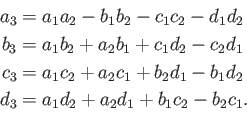(3.33)

In other words,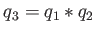as defined in (3.33).

Here is a way to rotate the point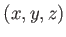using the rotation represented by. Let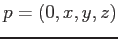, which is done to give the point the same dimensions as a quaternion. Perhaps surprisingly, the point is rotated by applying quaternion multiplication as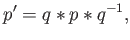(3.34)

in which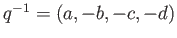(recall from Figure 3.12). The rotated point is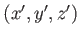, which is taken from the result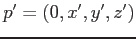.

Here is a simple example for the point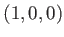. Let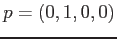and consider executing a yaw rotation by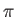. According to Figure 3.11, the corresponding quaternion is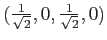. The inverse is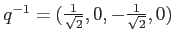. After tediously applying (3.33) to calculate (3.34), the result is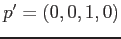. Thus, the rotated point is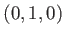, which is a correct yaw by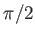.

Steven M LaValle 2020-11-11